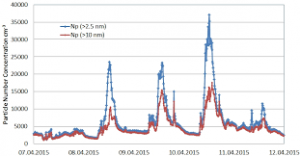## How to Calculate and Solve for the Volume of a Constituent, Volume of a Mixture and Volume Concentration in Chemistry | The Calculator EncyclopediaThe image above represents the volume concentration.

To compute the volume concentration of a substance, two essential parameters are needed and these parameters are volume of the constituent (Vi) and volume of the mixture (V).

The formula for calculating volume concentration:

φ = ViV

Where;
φ = Volume concentration
Vi = Volume of the constituent
V = Volume of the mixture

Let’s solve an example;
Find the volume concentration with a volume of the constituent of 23 and a volume of the mixture of 32.

This implies that;
Vi = Volume of the constituent = 23
V = Volume of the mixture = 32

φ = ViV
φ = 2332
φ = 0.718

Therefore, the volume concentration is 0.718.

Calculating the Volume of the Constituent when the Volume Concentration and the Volume of the Mixture is Given.

Vi = Vφ

Where;
φ = Volume concentration
V = Volume of the mixture

Let’s solve an example;
Find the volume of the constituent with a volume concentration of 18 and a volume of the mixture of 22.

This implies that;
φ = Volume concentration = 18
V = Volume of the mixture = 22

Vi = Vφ
Vi = 18 x 22
Vi = 396

Therefore, the volume of the constituent is 396 cm3.